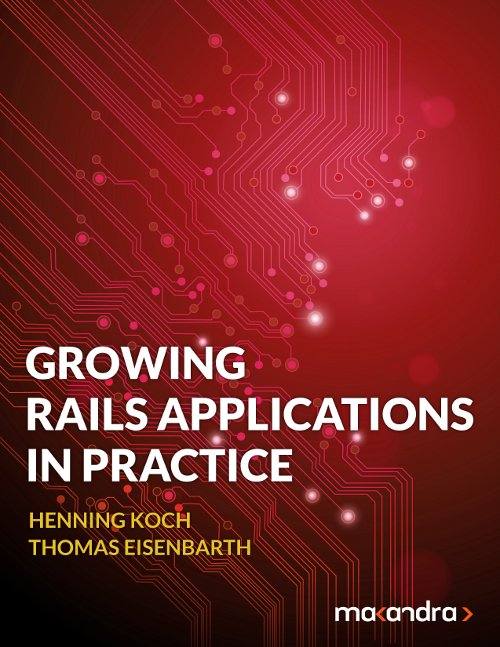Posted almost 10 years ago. Visible to the public. Repeats.

# Test if two date ranges overlap in Ruby or Rails

A check if two date or time ranges A and B overlap needs to cover a lot of cases:

1. A partially overlaps B

2. A surrounds B

3. B surrounds A

4. A occurs entirely after B

5. B occurs entirely after A

This means you actually have to check that:

• neither does A occur entirely after B (meaning `A.start > B.end`)
• nor does B occur entirely after A (meaning `B.start > A.end`)

Flipping this, A and B overlap iff `A.start <= B.end && B.start <= A.end`

The code below shows how to implement this in Ruby on Rails. The example is a class `Interval`, which has two attributes `#start_date` and `#end_date`. These dates are considered inclusive, meaning that if one interval ends on the day another interval starts, we consider those two intervals overlapping.

Note how we implemented both a version for loaded Ruby objects (`Interval#overlaps?`) and a scope that returns all overlapping intervals (`Interval.overlapping`). Depending on your problem you might need one or both or those.

```Copy```class Interval < ActiveRecord::Base

validates_presence_of :start_date, :end_date

# Check if a given interval overlaps this interval
def overlaps?(other)
start_date <= other.end_date && other.start_date <= end_date
end

# Return a scope for all interval overlapping the given interval, excluding the given interval itself
scope :overlapping, -> { |interval|
where("id <> ? AND start_date <= ? AND ? <= end_date", interval.id, interval.end_date, interval.start_date)
}

end
``````

This assumes that `start_date <= end_date` is always true.

## Mixing dates and times

If you mix dates and times incomparisons, mind to not compare datetimes with date ranges in MySQL.

## Visualisation

```Copy```
1. ------- -------
AAA       AAA
BBB     BBB
------- -------

2. -------
AAA
B
-------

3. -------
A
BBB
-------

4. -------
AAA
BBB
-------

5. -------
AAA
BBB
------

``````Check out our new e-book:
Learn to structure large Ruby on Rails codebases with the tools you already know and love.
Last edit:
over 2 years ago
by Emanuel De
Keywords:
time, activerecord, start_time, end_time